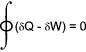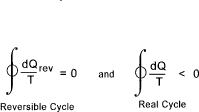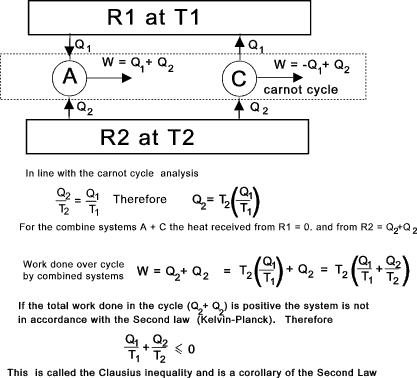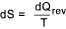Thermos Index

Laws of Thermodynamics

Introduction

The three laws of thermodynamics as noted below are very simple relatively obvious statements based on observations of the laws of nature.  They are however of fundamental importance to all physical and chemical processes.

Zeroth Law of Thermodynamics...

When two objects are separately in thermodynamic equilibrium with a third they are in equilibrium with each other.

Objects in thermodynamic equilibrium are at the same temperature.

The measuring of temperature with thermometers is really in accordance with this law.

First Law of Thermodynamics...

This law expresses the general law of conservation of energy. and states that heat and work are mutually convertible

Heat In = Work Out over complete cycle
or Sum (d Q ) = sum (d W )

Over a complete cycle the algebraic sum of the quantities of heat supplied to a system is equal to the algebraic sum quantities of work performed by the system i.e.In a cyclic process any property of the system are the same at the end of a cycle as at the beginning.  Throughout the path of a cycle (δQ - δW) represents a change in the total stored internal energy property of the system δE .   The basic energy equation results from this

δQ = δE + δW

The total stored internal energy E includes for various forms of energy including

 the various forms of potential energy (gravity, magnetic, electrical) thermal energy chemical energy atomic energy kinetic energy surface tension energy

Note: In classical thermodynamics as applicable to mechanical engineering the atomic energy and the chemical energy are not considered....

E = U + P.E + K.E + S.E

P.E = total potential energy, K.E = total kinetic energy, S.E = total surface energy .   The intrinsic internal energy U is the total internal energy minus the energies of motion, gravitational, magnetic and surface forces energies .  The first law can be written using U as

δQ = δU + δW

This is termed the restricted energy conservation equation for a system.   U is dependent on temperature and is not dependent on pressure or volume.

Examples of various non flow processes using the restricted energy conservation equation Non-Flow Processes

Second Law of Thermodynamics...

This law is derived from the whole field of physical experience.  Although it is not possible to prove the law or deduce it from other laws , no exception to it has yet been found.

This law in its simplest states that heat can only flow from hot to cold and not vice versa.  In terms of real thermodynamic engine cycles the law states that the gross heat supplied to a system in a complete cycle must exceed the work done by the system.   Therefore heat must be rejected.  The thermal efficiency of an heat engine must be less than 100%.   The maximum theoretical efficiency achievable is by use of the Carnot cycle.  This is based on reversible cycles using ideal gases ref Carnot Cycle

There are a number of sources for this law each providing a different interpretation.

 Clausius - No system or device may operate so that the only process is the heat transfer from a cooler to a hotter body Planck - It is impossible to make an engine cycle which will use only one heat reservoir and thence convert all the heat into work Kelvin - If a body is at a temperature below that of its surroundings none of it heat may be transferred into work unaided.

Some simple conclusions resulting from this law are..

 Work and Heat are mutually interchangeable(First law) but not completely (Second Law). A refrigerator cannot be designed to cool below the ambient temperature without use of an additional energy source A real heat engine can only be made to operate if there is a heat source and a heat sink
Clausius Inequality

The Clausius inequality is a corollary of the second law and states that if a system completes a cycle then.This principle can be tested by considering a system A which completes a complete cycle receiving heat Q1 from heat source R1 and heat Q2 from source R2.  In accordance with the first law the work done by A over a complete cycle = Q1 + Q2.  This system can now be isolated from the heat sources.   A second system C is provided which then completes a Carnot cycle which is arranged to reject Q1 to the heat source R1 and receive heat Q2from R2.  The carnot engine can be adjusted to match the conditions by setting of the isotherms and if necessary by having multiple cycles

The notes below show that for a simple system the Clausius Inequality conforms to the Second Law of thermodynamics.

The case of a more complicated cycle (A) with variable temperatures and consequent heat flows over the cycle is simply dealt with by using more and more matching Carnot cycles i.e. infinite of matching Carnot cycles with dQ heat transfer...Entropy

The value (dQ/T ) is a measure of the value of a property of the system called the entropy.  Entropy is defined asNotes on entropy are found on webpage Entropy

 Thermodynamic Law Links Thermodynamics..NASA - Glenn Research center at Series of informative notes on Thermodynamics Thermodynaic properties,property relationships and processes..A very detailed clear study of the subject, (3,3 Mbyte download) Laws of Thermodynamics..Useful notes links and notes on the subject

Thermos Index